Updating search results...

# 135 Results

View
Selected filters:
• NSDLUnrestricted Use
CC BY
Rating
0.0 stars

This task examines the ways in which the plane can be covered by regular polygons in a very strict arrangement called a regular tessellation. These tessellations are studied here using algebra, which enters the picture via the formula for the measure of the interior angles of a regular polygon (which should therefore be introduced or reviewed before beginning the task). The goal of the task is to use algebra in order to understand which tessellations of the plane with regular polygons are possible.

Subject:
Mathematics
Algebra
Geometry
Material Type:
Activity/Lab
Provider:
Illustrative Mathematics
Provider Set:
Illustrative Mathematics
Author:
Illustrative Mathematics
01/21/2013Conditional Remix & Share Permitted
CC BY-NC-SA
Rating
0.0 stars

In this activity, learners use a hand-made protractor to measure angles they find in playground equipment. Learners will observe that angle measurements do not change with distance, because they are distance invariant, or constant. Note: The "Pocket Protractor" activity should be done ahead as a separate activity (see related resource), but a standard protractor can be used as a substitute.

Subject:
Geometry
Material Type:
Activity/Lab
Provider:
Exploratorium
Author:
Exploratorium
Gordon and Betty Moore Foundation
National Science Foundation
The Exploratorium
12/07/2010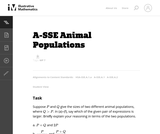Unrestricted Use
CC BY
Rating
0.0 stars

In this task students have to interpret expressions involving two variables in the context of a real world situation. All given expressions can be interpreted as quantities that one might study when looking at two animal populations.

Subject:
Mathematics
Algebra
Material Type:
Activity/Lab
Provider:
Illustrative Mathematics
Provider Set:
Illustrative Mathematics
Author:
Illustrative Mathematics
05/01/2012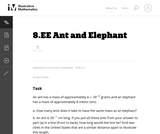Unrestricted Use
CC BY
Rating
0.0 stars

In this problem students are comparing a very small quantity with a very large quantity using the metric system. The metric system is especially convenient when comparing measurements using scientific notations since different units within the system are related by powers of ten.

Subject:
Mathematics
Material Type:
Activity/Lab
Provider:
Illustrative Mathematics
Provider Set:
Illustrative Mathematics
Author:
Illustrative Mathematics
05/01/2012Unrestricted Use
CC BY
Rating
0.0 stars

This task requires students to work with very large and small values expressed both in scientific notation and in decimal notation (standard form). In addition, students need to convert units of mass.

Subject:
Mathematics
Material Type:
Activity/Lab
Provider:
Illustrative Mathematics
Provider Set:
Illustrative Mathematics
Author:
Illustrative Mathematics
08/21/2012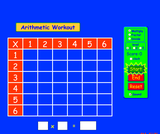Unrestricted Use
CC BY
Rating
5.0 stars

Brush up on your multiplication, division, and factoring skills with this interactive multiplication chart. Three levels and timed or untimed options are available.

Subject:
Mathematics
Material Type:
Simulation
Provider:
Provider Set:
PhET Interactive Simulations
Author:
Michael Dubson
11/05/2011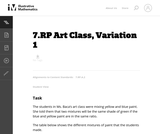Unrestricted Use
CC BY
Rating
0.0 stars

This this task about mixing paint requires students to graph ratios on a coordinate plane. It is a standard language in ratio problem.

Subject:
Mathematics
Material Type:
Activity/Lab
Provider:
Illustrative Mathematics
Provider Set:
Illustrative Mathematics
Author:
Illustrative Mathematics
05/01/2012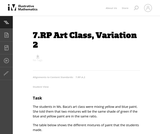Unrestricted Use
CC BY
Rating
0.0 stars

This this task about mixing paint requires students to graph ratios on a coordinate plane. It is a standard language in ratio problem.

Subject:
Mathematics
Material Type:
Activity/Lab
Provider:
Illustrative Mathematics
Provider Set:
Illustrative Mathematics
Author:
Illustrative Mathematics
05/01/2012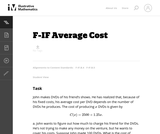Unrestricted Use
CC BY
Rating
0.0 stars

In this real world problem students solve questions based on the relationship between production costs and price.

Subject:
Mathematics
Functions
Material Type:
Activity/Lab
Provider:
Illustrative Mathematics
Provider Set:
Illustrative Mathematics
Author:
Illustrative Mathematics
05/01/2012Unrestricted Use
CC BY
Rating
0.0 stars

This task provides a real world context for interpreting and solving exponential equations. There are two solutions provided for part (a). The first solution demonstrates how to deduce the conclusion by thinking in terms of the functions and their rates of change. The second approach illustrates a rigorous algebraic demonstration that the two populations can never be equal.

Subject:
Mathematics
Functions
Material Type:
Activity/Lab
Provider:
Illustrative Mathematics
Provider Set:
Illustrative Mathematics
Author:
Illustrative Mathematics
05/01/2012Unrestricted Use
CC BY
Rating
0.0 stars

Subject:
Mathematics
Algebra
Material Type:
Activity/Lab
Provider:
Illustrative Mathematics
Provider Set:
Illustrative Mathematics
Author:
Illustrative Mathematics
05/01/2012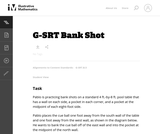Unrestricted Use
CC BY
Rating
0.0 stars

This task asks students to use similarity to solve a problem in a context that will be familiar to many, though most students are accustomed to using intuition rather than geometric reasoning to set up the shot.

Subject:
Mathematics
Geometry
Trigonometry
Material Type:
Activity/Lab
Provider:
Illustrative Mathematics
Provider Set:
Illustrative Mathematics
Author:
Illustrative Mathematics
08/21/2012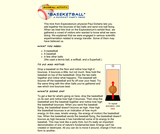Conditional Remix & Share Permitted
CC BY-NC-SA
Rating
0.0 stars

This trick from Exploratorium physicist Paul Doherty lets you add together the bounces of two balls and send one ball flying. When we tried this trick on the Exploratorium's exhibit floor, we gathered a crowd of visitors who wanted to know what we were doing. We explained that we were engaged in serious scientific experimentation related to energy transfer. Some of them may have believed us. If you'd like to go into the physical calculations of this phenomenam, see the related resource "Bouncing Balls" - it's the same activity but with the math explained.

Subject:
Physics
Material Type:
Activity/Lab
Provider:
Exploratorium
Author:
Paul Doherty
The Exploratorium
11/07/2012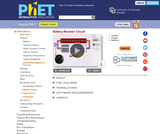Unrestricted Use
CC BY
Rating
0.0 stars

Look inside a resistor to see how it works. Increase the battery voltage to make more electrons flow though the resistor. Increase the resistance to block the flow of electrons. Watch the current and resistor temperature change.

Subject:
Physics
Material Type:
Simulation
Provider:
Provider Set:
PhET Interactive Simulations
Author:
Carl Wieman
Sam Reid
11/20/2008Only Sharing Permitted
CC BY-NC-ND
Rating
0.0 stars

This activity explores the main algorithms that are used as the basis for searching on computers, using different variations on the game of battleships. This activity demonstrates three search methods for finding information in data: linear searching, binary searching and hashing. It also includes an optional introductory activity as well as a video showing a fun demonstration related to the same content.

Subject:
Computer Science
Engineering
Education
Mathematics
Geometry
Material Type:
Activity/Lab
Game
Lesson Plan
Simulation
Provider:
Author:
Brian Mason Scientific and Technical Trust
Computer Science Unplugged
Ian Witten
Jane McKenzie
Mike Fellows
Tim Bell
01/02/2002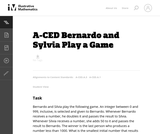Unrestricted Use
CC BY
Rating
0.0 stars

This task presents a simple but mathematically interesting game whose solution is a challenging exercise in creating and reasoning with algebraic inequalities. The core of the task involves converting a verbal statement into a mathematical inequality in a context in which the inequality is not obviously presented, and then repeatedly using the inequality to deduce information about the structure of the game.

Subject:
Mathematics
Algebra
Material Type:
Activity/Lab
Provider:
Illustrative Mathematics
Provider Set:
Illustrative Mathematics
Author:
Illustrative Mathematics
10/21/2013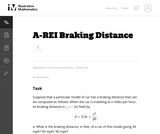Unrestricted Use
CC BY
Rating
0.0 stars

This task provides an exploration of a quadratic equation by descriptive, numerical, graphical, and algebraic techniques. Based on its real-world applicability, teachers could use the task as a way to introduce and motivate algebraic techniques like completing the square, en route to a derivation of the quadratic formula.

Subject:
Mathematics
Algebra
Functions
Material Type:
Activity/Lab
Provider:
Illustrative Mathematics
Provider Set:
Illustrative Mathematics
Author:
Illustrative Mathematics
05/01/2012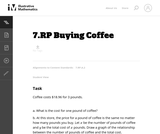Unrestricted Use
CC BY
Rating
0.0 stars

This is a task where it would be appropriate for students to use technology such as a graphing calculator or GeoGebra, making it a good candidate for students to engage in Standard for Mathematical Practice 5 Use appropriate tools strategically.

Subject:
Mathematics
Material Type:
Activity/Lab
Provider:
Illustrative Mathematics
Provider Set:
Illustrative Mathematics
Author:
Illustrative Mathematics
05/01/2012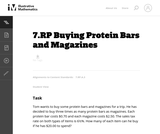Unrestricted Use
CC BY
Rating
0.0 stars

This task requires students to solves multistep ratio and percent problem.

Subject:
Mathematics
Material Type:
Activity/Lab
Provider:
Illustrative Mathematics
Provider Set:
Illustrative Mathematics
Author:
Illustrative Mathematics
05/01/2012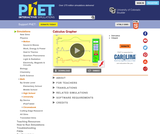Unrestricted Use
CC BY
Rating
0.0 stars

Draw a graph of any function and see graphs of its derivative and integral. Don't forget to use the magnify/demagnify controls on the y-axis to adjust the scale.

Subject:
Calculus
Material Type:
Simulation
Provider: